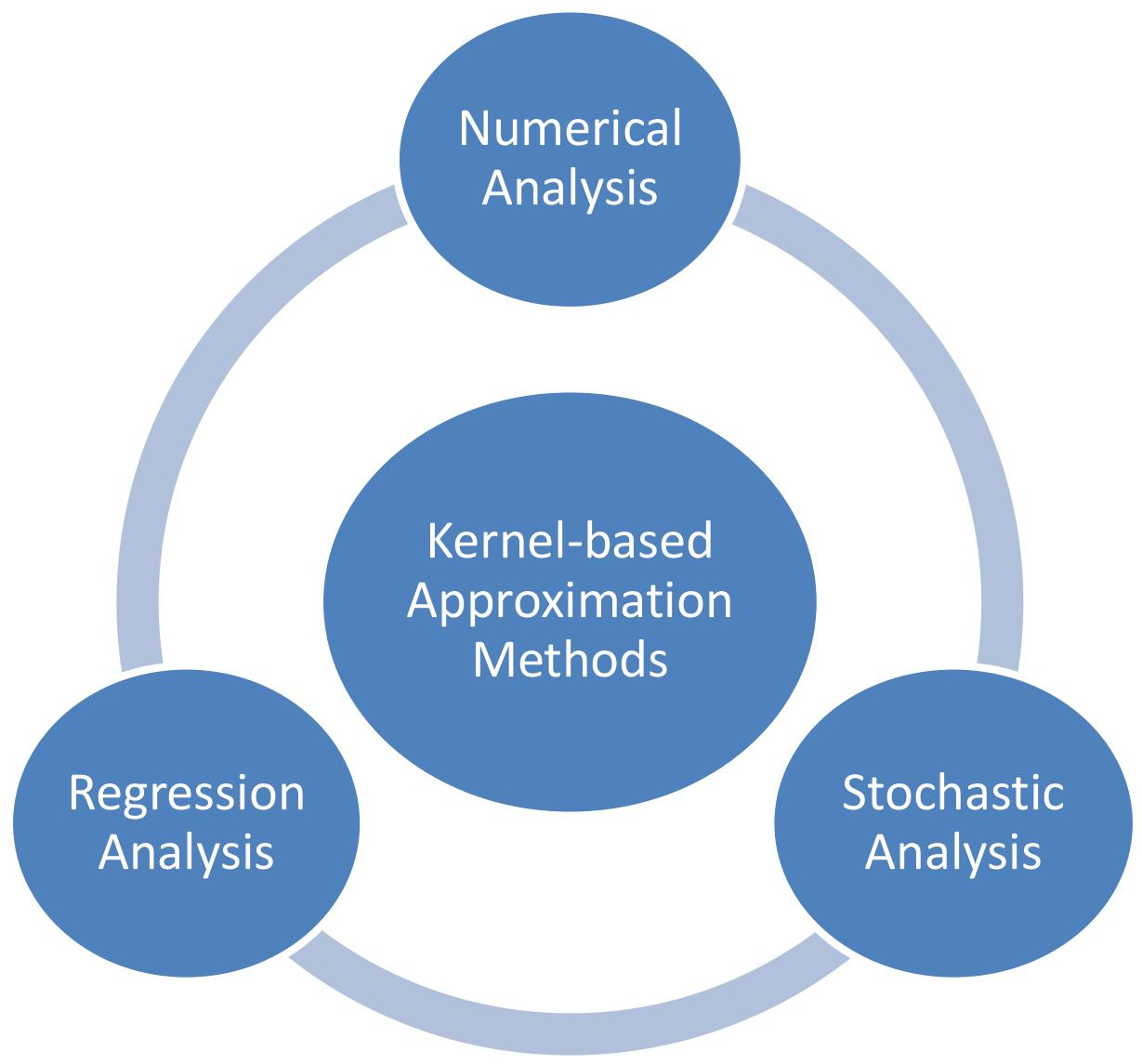# Optimal designs of postive definite kernels for scattered data approximation

In the research, we study the optimal designs of the positive definite kernels for the high-dimensional interpolation. We endow the Sobolev spaces with the probability measures induced by the positive definite kernels such that the kernel-based estimators can be solved to maximize the kernel-based probabilities conditioned on the observed data. In the practical implementations, we have many choices of the positive definite kernels to construct the kernel basis such as the Gaussian kernels with various shape parameters; hence we have an open problem what the optimal kernels are. The kernel-based probabilities provide a novel way to search the optimal kernels for the observed data. Combining with the statistical techniques such as the maximum likelihood estimation, we can solve the optimal shape parameters of the Gaussian kernels by the kernel-based probabilities even though the classical kernel-based methods cannot achieve the uncertain data.1、Qi Ye. Optimal designs of positive definite kernels for scattered data approximation. Applied and Computational Harmonic Analysis, 41(1), 214-236, 2016. DOI: 10.1016/j.acha.2015.08.009